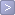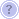Mortgage calculators  /  APR calculator

 To find out the annual percentage rate of your loan, enter the loan amount, interest rate, points, other costs and year-length term into the boxes below.

APR calculator
Loan Amount: \$Interest Rate: %Loan Term: yearsPoints:Other Costs: \$